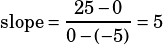The parabola above is the graph of y= -x^2 + k, where k is a constant.
If AB=10, what is the slope of AP?

A) 2
B) 5/2
C) 5
D) 10
E) 20

You know the parabola’s axis of symmetry is the y-axis, so you know that points A and B are (–5, 0) and (5, 0), respectively. Let’s use one of those to solve for k:

0 = –(5^2) + k
0 = –25 + k
25 = k

Now that you know k, you can use what you know about graph translation to see quickly that point P is (0, 25). So find the slope between (–5, 0) and (0, 25):